In :
options(repr.plot.width=5, repr.plot.height=5)


## Diagnostics in multiple linear regression¶

### Outline¶

• Diagnostics – again

• Different types of residuals

• Influence

• Outlier detection

• Residual plots:

• partial regression (added variable) plot,

• partial residual (residual plus component) plot.

## Scottish hill races data¶

The dataset we will use is based on record times on Scottish hill races.

 Variable Description Time Record time to complete course Distance Distance in the course Climb Vertical climb in the course
In :
url = 'http://www.statsci.org/data/general/hills.txt'

RaceDistanceClimbTime
Greenmantle2.5 650 16.083
Carnethy 6.0 2500 48.350
CraigDunain6.0 900 33.650
BenRha 7.5 800 45.600
BenLomond 8.0 3070 62.267
Goatfell 8.0 2866 73.217

As we'd expect, the time increases both with Distance and Climb.

In :
plot(races.table[,2:4], pch=23, bg='orange', cex=2)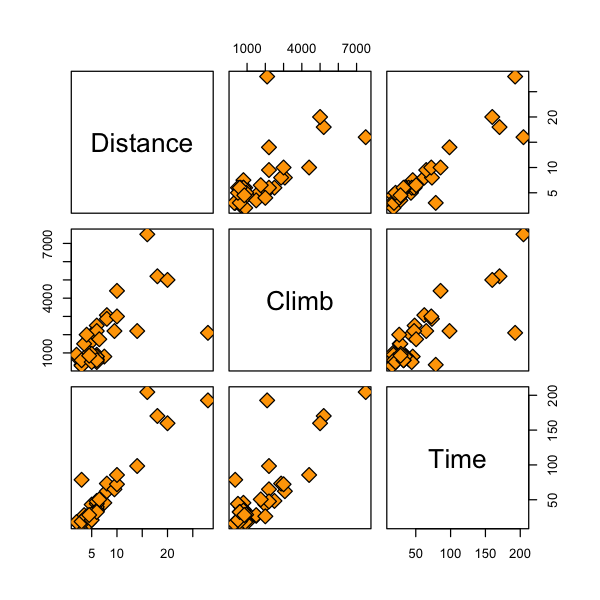Let's look at our multiple regression model.

In :
races.lm = lm(Time ~ Distance + Climb, data=races.table)
summary(races.lm)

Call:
lm(formula = Time ~ Distance + Climb, data = races.table)

Residuals:
Min      1Q  Median      3Q     Max
-16.215  -7.129  -1.186   2.371  65.121

Coefficients:
Estimate Std. Error t value Pr(>|t|)
(Intercept) -8.992039   4.302734  -2.090   0.0447 *
Distance     6.217956   0.601148  10.343 9.86e-12 ***
Climb        0.011048   0.002051   5.387 6.45e-06 ***
---
Signif. codes:  0 ‘***’ 0.001 ‘**’ 0.01 ‘*’ 0.05 ‘.’ 0.1 ‘ ’ 1

Residual standard error: 14.68 on 32 degrees of freedom
Multiple R-squared:  0.9191,	Adjusted R-squared:  0.914
F-statistic: 181.7 on 2 and 32 DF,  p-value: < 2.2e-16


But is this a good model?

## Diagnostics¶

### What can go wrong?¶

• Regression function can be wrong: maybe regression function should have some other form (see diagnostics for simple linear regression).

• Model for the errors may be incorrect:

• may not be normally distributed.

• may not be independent.

• may not have the same variance.

• Detecting problems is more art then science, i.e. we cannot test for all possible problems in a regression model.

## Diagnostics¶

• Basic idea of diagnostic measures: if model is correct then residuals $e_i = Y_i -\widehat{Y}_i, 1 \leq i \leq n$ should look like a sample of (not quite independent) $N(0, \sigma^2)$ random variables.

## Standard diagnostic plots¶

R produces a set of standard plots for lm that help us assess whether our assumptions are reasonable or not. We will go through each in some, but not too much, detail.

As we see below, there are some quantities which we need to define in order to read these plots. We will define these first.

In :
par(mfrow=c(2,2))
plot(races.lm, pch=23 ,bg='orange',cex=2)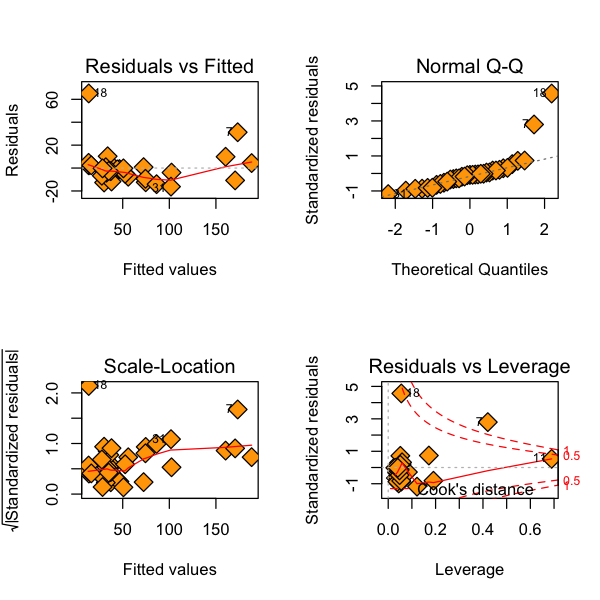## Problems with the errors¶

### Possible problems & diagnostic checks¶

• Errors may not be normally distributed or may not have the same variance – qqnorm can help with this. This may not be too important in large samples.

• Variance may not be constant. Can also be addressed in a plot of $X$ vs. $e$ : fan shape or other trend indicate non-constant variance.

• Influential observations. Which points “affect” the regression line the most?

• Outliers: points where the model really does not fit! Possibly mistakes in data transcription, lab errors, who knows? Should be recognized and (hopefully) explained.

## Types of residuals¶

• Ordinary residuals: $e_i = Y_i - \widehat{Y}_i$. These measure the deviation of predicted value from observed value, but their distribution depends on unknown scale, $\sigma$.

• Internally studentized residuals (rstandard in R): $$r_i = e_i / SE(e_i) = \frac{e_i}{\widehat{\sigma} \sqrt{1 - H_{ii}}}$$

• Above, $H$ is the “hat” matrix $H=X(X^TX)^{-1}X^T$. These are almost $t$-distributed, except $\widehat{\sigma}$ depends on $e_i$.

## Types of residuals¶

• Externally studentized residuals (rstudent in R): $$t_i = \frac{e_i}{\widehat{\sigma_{(i)}} \sqrt{1 - H_{ii}}} \sim t_{n-p-2}.$$ These are exactly $t$ distributed so we know their distribution and can use them for tests, if desired.

• The quantity $\hat{\sigma}^2_{(i)}$ is the MSE of the model fit to all data except case $i$ (i.e. it has $n-1$ observations and $p$ features).

• Numerically, these residuals are highly correlated, as we would expect.

In :
plot(resid(races.lm), rstudent(races.lm), pch=23, bg='blue', cex=3)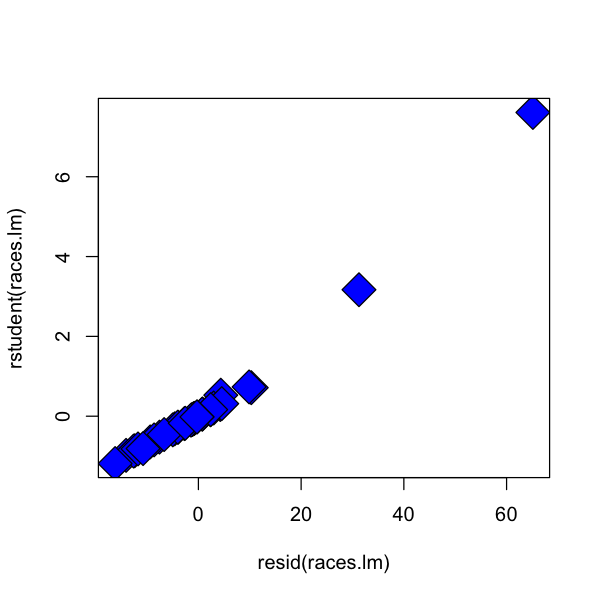In :
plot(rstandard(races.lm), rstudent(races.lm), pch=23, bg='blue', cex=3)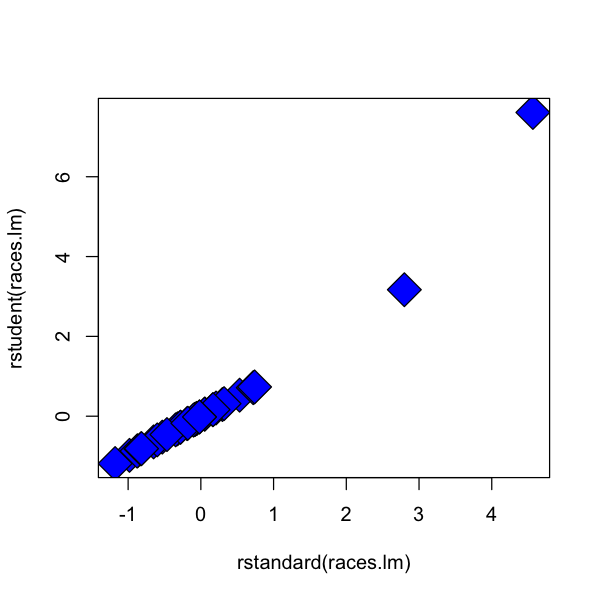## Standard diagnostic plots¶

The first plot is the quantile plot for the residuals, that compares their distribution to that of a sample of independent normals.

In :
qqnorm(rstandard(races.lm), pch=23, bg='red', cex=2)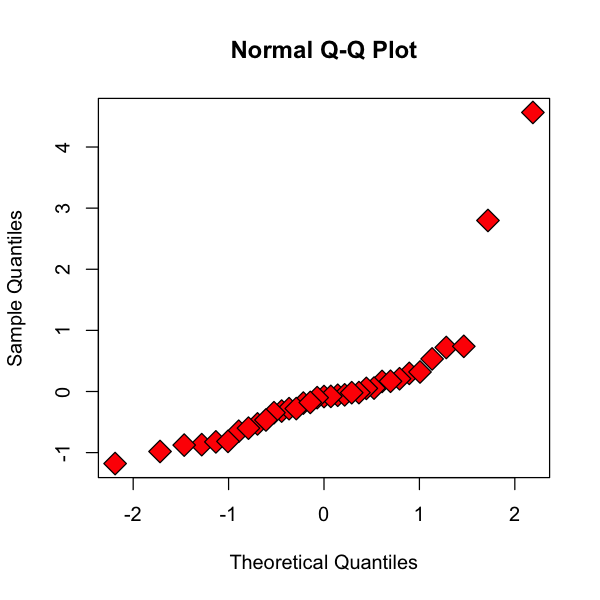If the residuals were really normal we'd expect this plot to be roughly on the diagonal.

In :
qqnorm(rnorm(500), pch=23, bg='red', cex=2)
abline(0, 1)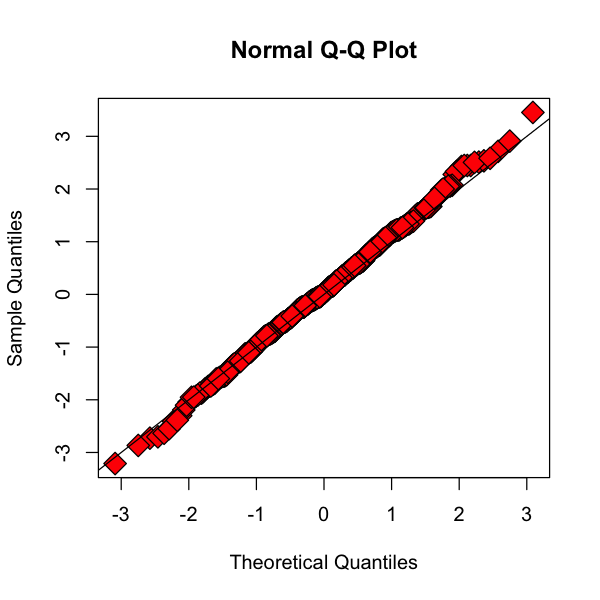Two other plots try address the constant variance assumptions. If these plots have a particular shape (maybe the spread increases with $\hat{Y}$) then maybe the variance is not constant.

In :
plot(fitted(races.lm), sqrt(abs(rstandard(races.lm))), pch=23, bg='red', ylim=c(0,1))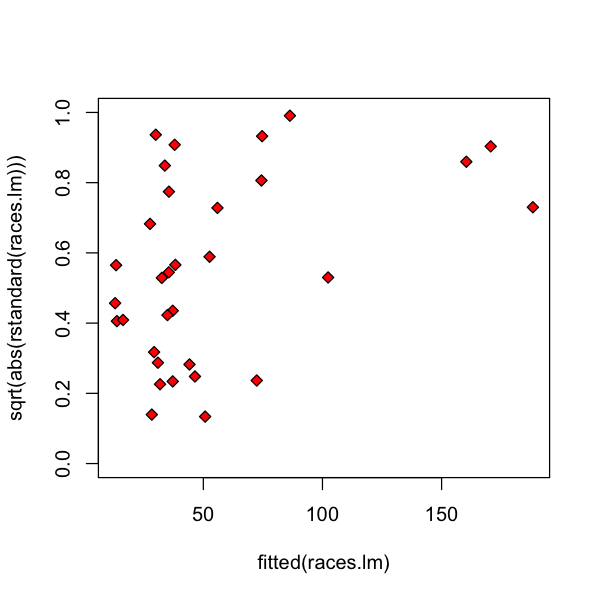In :
plot(fitted(races.lm), resid(races.lm), pch=23, bg='red', cex=2)
abline(h=0, lty=2)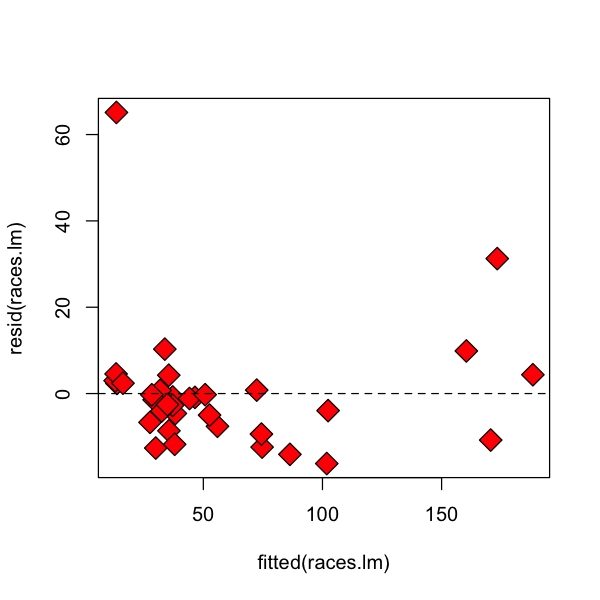## Influence of an observation¶

Other plots provide an assessment of the influence of each observation. Usually, this is done by dropping an entire case $(y_i, x_i)$ from the dataset and refitting the model.

• In this setting, a $\cdot_{(i)}$ indicates $i$-th observation was not used in fitting the model.

• For example: $\widehat{Y}_{j(i)}$ is the regression function evaluated at the $j$-th observation predictors BUT the coefficients $(\widehat{\beta}_{0(i)}, \dots, \widehat{\beta}_{p(i)})$ were fit after deleting $i$-th case from the data.

## Influence of an observation¶

• Idea: if $\widehat{Y}_{j(i)}$ is very different than $\widehat{Y}_j$ (using all the data) then $i$ is an influential point, at least for estimating the regression function at $(X_{1,j}, \dots, X_{p,j})$.

• Could also look at difference between $\widehat{Y}_{i(i)} - \widehat{Y}_i$, or any other measure.

• There are various standard measures of influence.

## DFFITS¶

• $$DFFITS_i = \frac{\widehat{Y}_i - \widehat{Y}_{i(i)}}{\widehat{\sigma}_{(i)} \sqrt{H_{ii}}}$$

• This quantity measures how much the regression function changes at the $i$-th case / observation when the $i$-th case / observation is deleted.

• For small/medium datasets: value of 1 or greater is “suspicious” (RABE). For large dataset: value of $2 \sqrt{(p+1)/n}$.

• R has its own standard rules similar to the above for marking an observation as influential.

In :
plot(dffits(races.lm), pch=23, bg='orange', cex=2, ylab="DFFITS")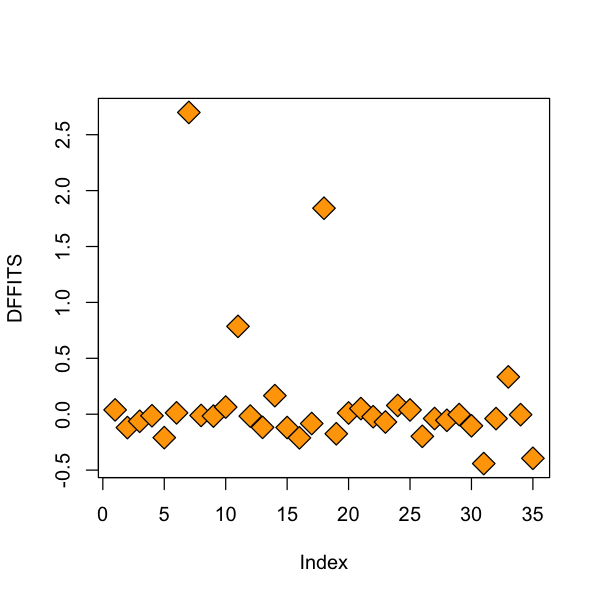It seems that some observations had a high influence measured by $DFFITS$:

In :
races.table[which(dffits(races.lm) > 0.5),]

RaceDistanceClimbTime
7BensofJura16 7500 204.617
11LairigGhru28 2100 192.667
18KnockHill 3 350 78.650

It is perhaps not surprising that the longest course and the course with the most elevation gain seemed to have a strong effect on the fitted values. What about Knock Hill? We'll come back to this later.

## Cook’s distance¶

Cook’s distance measures how much the entire regression function changes when the $i$-th case is deleted.

• $$D_i = \frac{\sum_{j=1}^n(\widehat{Y}_j - \widehat{Y}_{j(i)})^2}{(p+1) \, \widehat{\sigma}^2}$$

• Should be comparable to $F_{p+1,n-p-1}$: if the “$p$-value” of $D_i$ is 50 percent or more, then the $i$-th case is likely influential: investigate further. (RABE)

• Again, R has its own rules similar to the above for marking an observation as influential.

• What to do after investigation? No easy answer.

In :
plot(cooks.distance(races.lm), pch=23, bg='orange', cex=2, ylab="Cook's distance")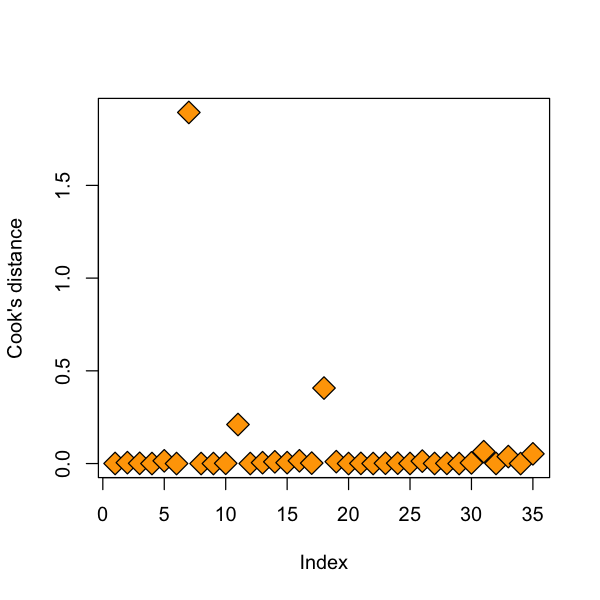In :
races.table[which(cooks.distance(races.lm) > 0.1),]

RaceDistanceClimbTime
7BensofJura16 7500 204.617
11LairigGhru28 2100 192.667
18KnockHill 3 350 78.650

Again, the same 3 races. This is not surprising as both $DFFITS$ and Cook's distance measure changes in fitted values. The difference is that one measures the influence on one fitted value, while the other measures the influence on the entire vector of fitted values.

## DFBETAS¶

This quantity measures how much the coefficients change when the $i$-th case is deleted.

• $$DFBETAS_{j(i)} = \frac{\widehat{\beta}_j - \widehat{\beta}_{j(i)}}{\sqrt{\widehat{\sigma}^2_{(i)} (X^TX)^{-1}_{jj}}}.$$
• For small/medium datasets: absolute value of 1 or greater is “suspicious”. For large dataset: absolute value of $2 / \sqrt{n}$.
In :
plot(dfbetas(races.lm)[,'Climb'], pch=23, bg='orange', cex=2, ylab="DFBETA (Climb)")
races.table[which(abs(dfbetas(races.lm)[,'Climb']) > 1),]

RaceDistanceClimbTime
7BensofJura16 7500 204.617In :
plot(dfbetas(races.lm)[,'Distance'], pch=23, bg='orange', cex=2, ylab="DFBETA (Climb)")
races.table[which(abs(dfbetas(races.lm)[,'Distance']) > 0.5),]

RaceDistanceClimbTime
7BensofJura16 7500 204.617
11LairigGhru28 2100 192.667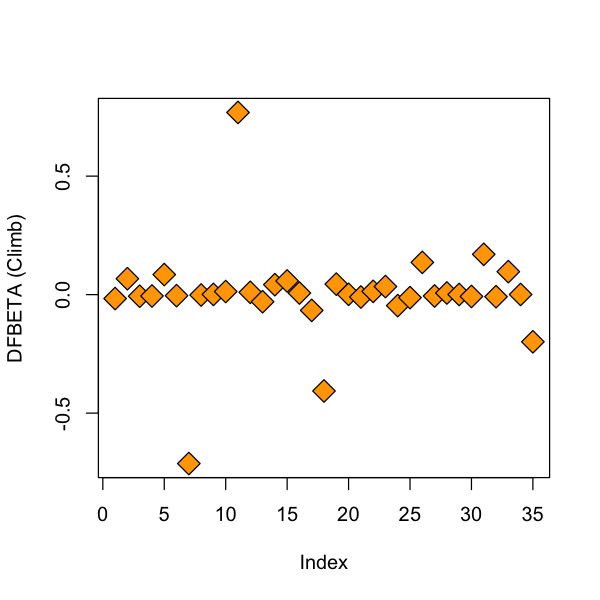## Outliers¶

The essential definition of an outlier is an observation pair $(Y, X_1, \dots, X_p)$ that does not follow the model, while most other observations seem to follow the model.

• Outlier in predictors: the $X$ values of the observation may lie outside the “cloud” of other $X$ values. This means you may be extrapolating your model inappropriately. The values $H_{ii}$ can be used to measure how “outlying” the $X$ values are.

• Outlier in response: the $Y$ value of the observation may lie very far from the fitted model. If the studentized residuals are large: observation may be an outlier.

• The races at Bens of Jura and Lairig Ghru seem to be outliers in predictors as they were the highest and longest races, respectively.

• How can we tell if the Knock Hill result is an outlier? It seems to have taken much longer than it should have so maybe it is an outlier in the response.

## Outlying $X$ values¶

One way to detect outliers in the predictors, besides just looking at the actual values themselves, is through their leverage values, defined by $$\text{leverage}_i = H_{ii} = (X(X^TX)^{-1}X^T)_{ii}.$$

Not surprisingly, our longest and highest courses show up again. This at least reassures us that the leverage is capturing some of this "outlying in $X$ space".

In :
plot(hatvalues(races.lm), pch=23, bg='orange', cex=2, ylab='Hat values')
races.table[which(hatvalues(races.lm) > 0.3),]

RaceDistanceClimbTime
7BensofJura16 7500 204.617
11LairigGhru28 2100 192.667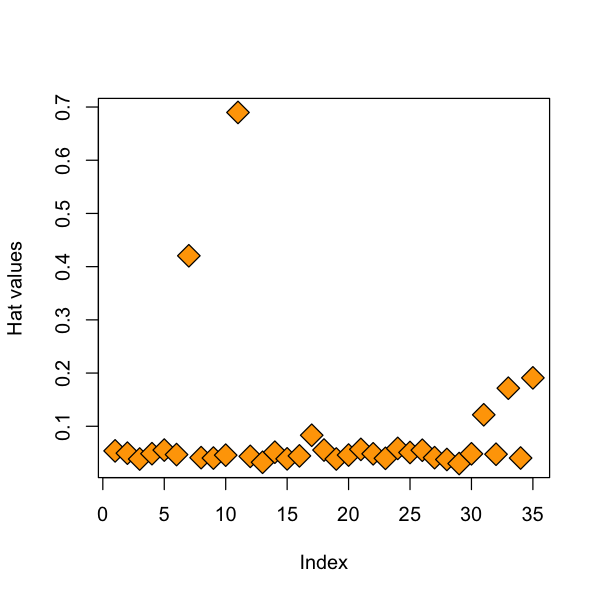## Outliers in the response¶

We will consider a crude outlier test that tries to find residuals that are "larger" than they should be.

• Since rstudent are $t$ distributed, we could just compare them to the $T$ distribution and reject if their absolute value is too large.

• Doing this for every observation results in $n$ different hypothesis tests.

• This causes a problem: if $n$ is large, if we “threshold” at $t_{1-\alpha/2, n-p-2}$ we will get many outliers by chance even if model is correct.

• In fact, we expect to see $n \cdot \alpha$ “outliers” by this test. Every large data set would have outliers in it, even if model was entirely correct!

Let's sample some data from our model to convince ourselves that this is a real problem.

In :
X = rnorm(100)
Y = 2 * X + 0.5 + rnorm(100)
alpha = 0.1
cutoff = qt(1 - alpha / 2, 97)
sum(abs(rstudent(lm(Y~X))) > cutoff)

11
In :
# Bonferroni correction
X = rnorm(100)
Y = 2 * X + 0.5 + rnorm(100)
cutoff = qt(1 - (alpha / 100) / 2, 97)
sum(abs(rstudent(lm(Y~X))) > cutoff)

0

### Multiple comparisons¶

• This problem we identified is known as multiple comparisons or simultaneous inference.

• When performing many tests (say $m$) each at level $\alpha$, we expect at least $\alpha m$ rejections even when all null hypotheses are true!

• In outlier detection, we are performing $m=n$ hypothesis tests, but might still like to control the probability of making any false positive errors.

• The reason we don't want to make errors here is that we don't want to throw away data unnecessarily.

• One solution: Bonferroni correction, threshold at $t_{1 - \alpha/(2*n), n-p-2}$.

### Bonferroni correction¶

• Dividing $\alpha$ by $n$, the number of tests, is known as a Bonferroni correction.

• If we are doing many $t$ (or other) tests, say $m \gg 1$ we can control overall false positive rate at $\alpha$ by testing each one at level $\alpha/m$.

• In this case $m=n$, but other times we might look at a different number of tests.

### Bonferroni correction¶

• Essentially the union bound for probability.

• Proof: when the model is correct, with studentized residuals $T_i$:

\begin{aligned}  P\left( \text{at least one false positive} \right) & = P \left(\cup_{i=1}^m |T_i| \geq t_{1 - \alpha/(2*m), n-p-2} \right) \\ & \leq \sum_{i=1}^m P \left( |T_i| \geq t_{1 - \alpha/(2*m), n-p-2} \right) \\ & = \sum_{i=1}^m \frac{\alpha}{m} = \alpha. \\ \end{aligned}

Let's apply this to our data. It turns out that KnockHill is a known error.

In :
n = nrow(races.table)
cutoff = qt(1 - 0.05 / (2*n), (n - 4))
races.table[which(abs(rstudent(races.lm)) > cutoff),]

RaceDistanceClimbTime
18KnockHill3 350 78.65

The package car has a built in function to do this test.

In :
library(car)
outlierTest(races.lm)

   rstudent unadjusted p-value Bonferonni p
18 7.610845         1.3973e-08   4.8905e-07

### Final plot¶

The last plot that R produces is a plot of residuals against leverage. Points that have high leverage and large residuals are particularly influential.

In :
plot(hatvalues(races.lm), rstandard(races.lm), pch=23, bg='red', cex=2)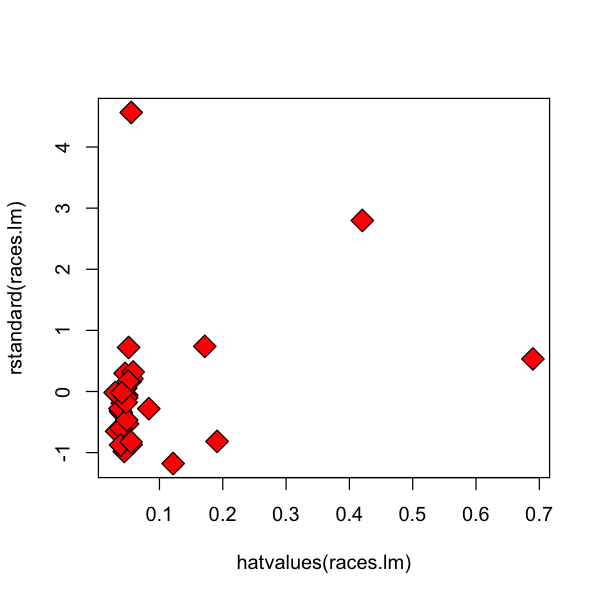R will put the IDs of cases that seem to be influential in these (and other plots). Not surprisingly, we see our usual three suspects.

In :
plot(races.lm, which=5)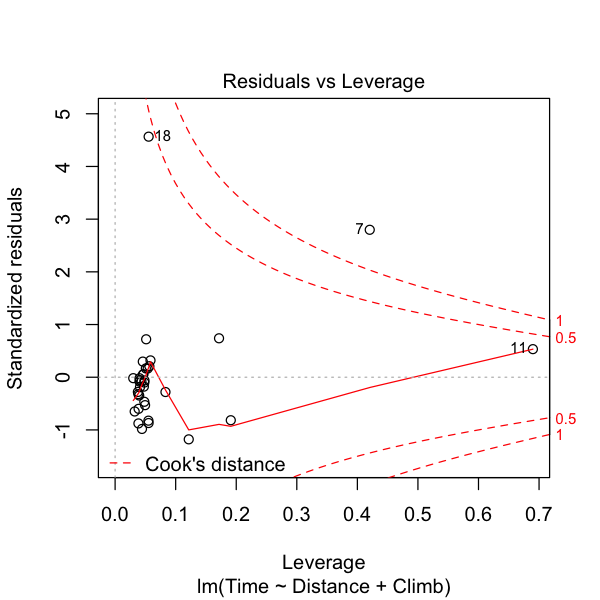## Influence measures¶

As mentioned above, R has its own rules for flagging points as being influential. To see a summary of these, one can use the influence.measures function.

In :
influence.measures(races.lm)

Influence measures of
lm(formula = Time ~ Distance + Climb, data = races.table) :

dfb.1_  dfb.Dstn  dfb.Clmb    dffit  cov.r   cook.d    hat inf
1   0.03781 -0.016614 -0.004744  0.03862 1.1595 5.13e-04 0.0538
2  -0.05958  0.067215 -0.073396 -0.11956 1.1269 4.88e-03 0.0495
3  -0.04858 -0.006707  0.028033 -0.06310 1.1329 1.37e-03 0.0384
4  -0.00766 -0.005675  0.008764 -0.01367 1.1556 6.43e-05 0.0485
5  -0.05046  0.084709 -0.145005 -0.20947 1.0837 1.47e-02 0.0553
6   0.00348 -0.004316  0.007576  0.01221 1.1536 5.13e-05 0.0468
7  -0.89065 -0.712774  2.364618  2.69909 0.8178 1.89e+00 0.4204   *
8  -0.00844 -0.001648  0.005562 -0.01115 1.1467 4.28e-05 0.0410
9  -0.01437  0.000913  0.006161 -0.01663 1.1453 9.52e-05 0.0403
10  0.04703  0.013057 -0.036519  0.06399 1.1431 1.41e-03 0.0457
11 -0.30118  0.768716 -0.479849  0.78569 3.4525 2.11e-01 0.6898   *
12 -0.01149  0.009656 -0.007488 -0.01672 1.1492 9.61e-05 0.0435
13 -0.03173 -0.029911 -0.000707 -0.11770 1.0922 4.70e-03 0.0323
14  0.11803  0.042034 -0.104884  0.16610 1.1039 9.34e-03 0.0513
15 -0.10038  0.057701 -0.022317 -0.11920 1.1062 4.83e-03 0.0388
16 -0.01852  0.006789 -0.099862 -0.21135 1.0501 1.49e-02 0.0444
17  0.01196 -0.066505  0.034455 -0.08337 1.1908 2.39e-03 0.0831
18  1.75827 -0.406545 -0.655934  1.84237 0.0493 4.07e-01 0.0554   *
19 -0.15889  0.044311  0.029414 -0.17484 1.0635 1.03e-02 0.0385
20  0.00866  0.001424 -0.005946  0.01102 1.1526 4.18e-05 0.0459
21  0.04777 -0.010019 -0.019199  0.05032 1.1611 8.70e-04 0.0566
22 -0.01889  0.013856 -0.006465 -0.02234 1.1546 1.72e-04 0.0483
23 -0.04131  0.034097 -0.033022 -0.06961 1.1326 1.66e-03 0.0398
24  0.07483 -0.046385  0.006428  0.07839 1.1571 2.11e-03 0.0584
25  0.03691 -0.012633 -0.008257  0.03808 1.1557 4.99e-04 0.0507
26 -0.13772  0.136124 -0.101306 -0.19782 1.0914 1.32e-02 0.0550
27 -0.02920 -0.005702  0.019239 -0.03857 1.1431 5.11e-04 0.0410
28 -0.04764  0.006936  0.014990 -0.05446 1.1345 1.02e-03 0.0376
29 -0.00214  0.000647 -0.000328 -0.00309 1.1338 3.29e-06 0.0299
30 -0.08532 -0.007705  0.054838 -0.10362 1.1323 3.67e-03 0.0482
31  0.02099  0.170124 -0.373634 -0.44138 1.0960 6.41e-02 0.1216
32 -0.02858 -0.008694  0.023275 -0.03931 1.1513 5.31e-04 0.0475
33 -0.15823  0.097014  0.155702  0.33384 1.2609 3.77e-02 0.1716
34 -0.00356  0.000704  0.001054 -0.00392 1.1461 5.29e-06 0.0403
35  0.20872 -0.199048 -0.100907 -0.39445 1.2764 5.24e-02 0.1910    

While not specified in the documentation, the meaning of the asterisks can be found by reading the code. The function is.influential makes the decisions to flag cases as influential or not.

• We see that the DFBETAS are thresholded at 1.

• We see that DFFITS is thresholded at 3 * sqrt((p+1)/(n-p-1)).

• Etc.

In :
influence.measures

function (model)
{
is.influential <- function(infmat, n) {
k <- ncol(infmat) - 4
if (n <= k)
stop("too few cases, n < k")
absmat <- abs(infmat)
result <- cbind(absmat[, 1L:k] > 1, absmat[, k + 1] >
3 * sqrt(k/(n - k)), abs(1 - infmat[, k + 2]) > (3 *
k)/(n - k), pf(infmat[, k + 3], k, n - k) > 0.5,
infmat[, k + 4] > (3 * k)/n)
dimnames(result) <- dimnames(infmat)
result
}
infl <- influence(model)
p <- model$rank e <- weighted.residuals(model) s <- sqrt(sum(e^2, na.rm = TRUE)/df.residual(model)) mqr <- qr.lm(model) xxi <- chol2inv(mqr$qr, mqr$rank) si <- infl$sigma
h <- infl$hat dfbetas <- infl$coefficients/outer(infl$sigma, sqrt(diag(xxi))) vn <- variable.names(model) vn[vn == "(Intercept)"] <- "1_" colnames(dfbetas) <- paste("dfb", abbreviate(vn), sep = ".") dffits <- e * sqrt(h)/(si * (1 - h)) if (any(ii <- is.infinite(dffits))) dffits[ii] <- NaN cov.ratio <- (si/s)^(2 * p)/(1 - h) cooks.d <- if (inherits(model, "glm")) (infl$pear.res/(1 - h))^2 * h/(summary(model)$dispersion * p) else ((e/(s * (1 - h)))^2 * h)/p infmat <- cbind(dfbetas, dffit = dffits, cov.r = cov.ratio, cook.d = cooks.d, hat = h) infmat[is.infinite(infmat)] <- NaN is.inf <- is.influential(infmat, sum(h > 0)) ans <- list(infmat = infmat, is.inf = is.inf, call = model$call)
class(ans) <- "infl"
ans
}

## Problems in the regression function¶

• True regression function may have higher-order non-linear terms, polynomial or otherwise.

• We may be missing terms involving more than one ${X}_{(\cdot)}$, i.e. ${X}_i \cdot {X}_j$ (called an interaction).

• Some simple plots: added-variable and component plus residual plots can help to find nonlinear functions of one variable.

• I find these plots of somewhat limited use in practice, but we will go over them as possibly useful diagnostic tools.

• The plots can be helpful for finding influential points, outliers. The functions can be found in the car package.

• Procedure:

• Let $\tilde{e}_{X_j,i}, 1\leq i \leq n$ be the residuals after regressing $X_j$ onto all columns of $X$ except $X_j$;

• Let $e_{X_j,i}$ be the residuals after regressing ${Y}$ onto all columns of ${X}$ except ${X}_j$;

• Plot $\tilde{e}_{X_j}$ against $e_{X_j}$.

• If the (partial regression) relationship is linear this plot should look linear.

In :
avPlots(races.lm, 'Distance')